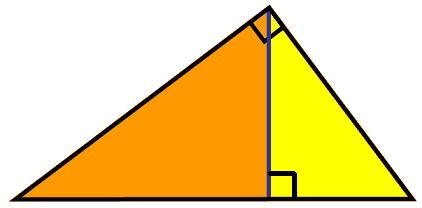#### You may also like### Fitting In

The largest square which fits into a circle is ABCD and EFGH is a square with G and H on the line CD and E and F on the circumference of the circle. Show that AB = 5EF. Similarly the largest equilateral triangle which fits into a circle is LMN and PQR is an equilateral triangle with P and Q on the line LM and R on the circumference of the circle. Show that LM = 3PQ### Look Before You Leap

Can you spot a cunning way to work out the missing length?### Triangle Midpoints

You are only given the three midpoints of the sides of a triangle. How can you construct the original triangle?

# Nicely Similar

##### Age 14 to 16Challenge Level

Nicely Similar printable sheet

In the diagram below, a right-angled triangle has a line descending from its right angle, perpendicular to the hypotenuse.If the hypotenuse length is 100cm, and if the line splits the base into 36cm and 64cm parts, what are the side lengths of the original right-angled triangle?

For another way to see this arrangement of right-angled triangles you may like to look at the problem called a Matter of Scale .